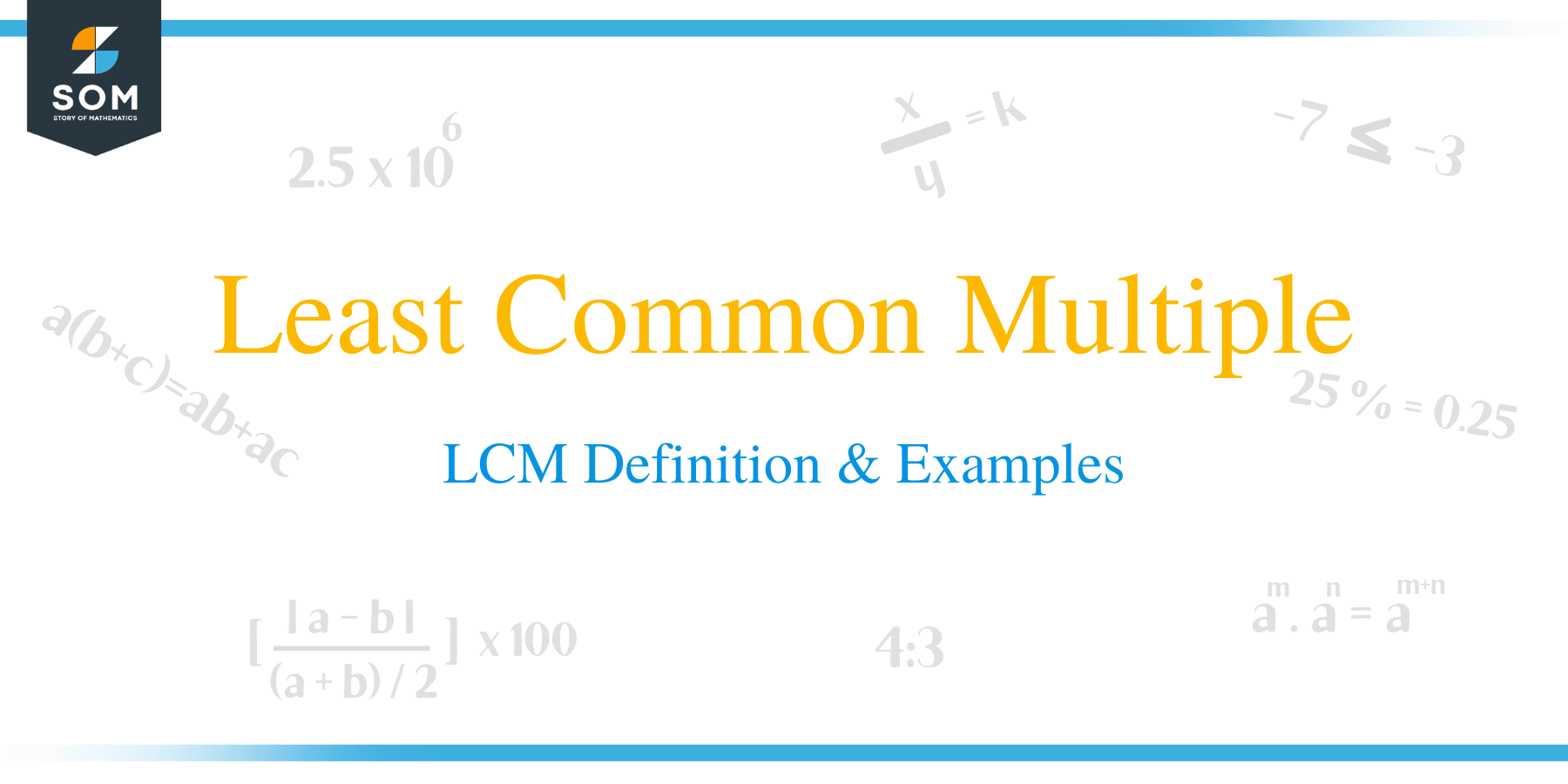# Least Common Multiple – LCM Definition & Examples

##What is a Least Common Multiple?

The least common multiple can be defined as the lowest positive integer that is multiple in a given set of numbers. The least common multiple is sometimes referred to as the lowest common multiple and abbreviated as (LCM).

For instance, the LCM of 2, 3, and 7 is 42 because 42 is a multiple of 2, 3, and 7. There is no other number lower than 42 that is a multiple of the three numbers.

## How to Find the Least Common Multiples?

The LCM of two or more numbers can be found by various methods. Some of these methods are explained below.

### Factorization method

The LCM of numbers can be calculated by factoring in all numbers in a set that is multiplied to generate that number as a product.

Example 1

Suppose you want to find the LCM of two numbers, 20 and 42.

Solution

• Begin by listing down factors of each number in the set.

20 = 2 x 2 x 5

42 = 2 x 3 x 7

• The LCM is obtained by multiplication of the factors of these number as:

2 x 2 x 3 x 5 x 7 = 420.

Example 2

Find the LCM of the set: 12, 15, and 18.

Solution

• Start by listing down the prime factors of each number:

12 = 2 x 2 x 3

15= 3 x 5

18 = 2 x 3 x 3

• Multiply the most repeated numbers as:

2 x 2 x 3 x 3 x 5 = 180

Example 3

Determine the LCM of 18 and 24 using the factorization method

Solution

• Write down the prime factors of each number in the set.

24 = 2 x 2 x 2 x 3

18 = 2 x 3 x 3

• Identify the most repeated number in each list.
• Since number 2 occurs once and thrice in 18 and 24, pick number 2 three times.
• Similarly, number 3 occurs once and twice in the list of 24 and 18, respectively, and so, pick number 3 twice.
• The product of the picked numbers gives the LCM of the numbers;
• LCM = 2 x 2 x 2 x 3 x 3 = 72

### Multiplication method

The LCM of numbers is found by listing down multiples of each number in the set. The first multiple to appear in both lists is taken to be the LCM of the set. It is explained in the example below.

Example 4

Find the LCM of 4 and 6 using the multiplication method

Solution

• Start by listing the multiples of both 4 and 6. Start with a higher number, and for this case, is 6.
• Multiples of 6 are: 6, 12, 18, 24, 30, …
• Multiples of 4 are: 4, 8, 12, . . .

The first common number to appear on the lists is 12; therefore, the LCM is 12.

This method is only suitable when finding the LCM of two numbers. If a set has more than two numbers, you can multiply two numbers in the set and work out the same way as with a set with two numbers.### Practice Questions

1. What is the least common multiple of $4$ and $10$?

2. What is the least common multiple of $7$ and $11$ using the multiplication method?

3. What is the least common multiple of $9$ and $12$?

4. What is the least common multiple of $18$ and $22$?

5. What is the least common multiple of $6$ and $15$ using the prime factor method?

6. What is the least common multiple of $4$, $6$, and $8$?

7. What is the least common multiple of $8$, $12$, and $18$?

8. What is the least common multiple of  $70$ and $90$?

9. What is the least common multiple of  $180$, $216$, and $450$?